﻿ 基于生化仪温度控制的电流检测系统设计

# 基于生化仪温度控制的电流检测系统设计 Design of Current Detection System Based on Temperature Control of Biochemical Instrument

Abstract: Based on the temperature control of the biochemical analyzer, a high-precision current detection system is designed in this paper. The design mainly includes the hardware circuit, software program and temperature control algorithm of the current detection system. The hardware content includes temperature and current detection circuit, refrigeration module, CAN communication module, display module. Use KEIL MDK software to develop the main control program of the current detection system. The temperature control algorithm adopts PID algorithm. Through multiple tests to verify the relevant parameters, the current detection system based on temperature control designed in the article can accurately calculate the current value and the instrument temperature value, and communicate with the upper computer (PC) in time and accurately. The temperature range designed in the article is controlled at 7˚C~13˚C, the measurement accuracy is ±0.1˚C, and the corresponding current value is about 3000 mA, the measurement accuracy is ±1 mA. The corresponding parameters can be adjusted for other occasions.

1. 引言

2. 电流检测系统总体方案设计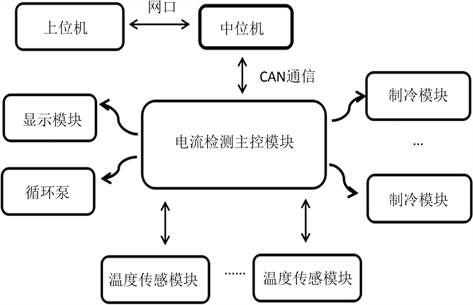Figure 1. Current detection system

3. 系统硬件单元设计

3.1. 电流检测MCU选取

STM32f103r8t6单片机  采用Cortex-M3内核，快速闪存记忆体高达128千字节和20字节SRAM，具备多种外设接口及先进高级的通讯接口。相比51系列、AVR系列单片机，该单片机功能强大且更加经济实惠、易于操作，拥有更高效的代码效率。本次设计，采用64 KB Flash，工作电压2.0~3.6 V，工作温度在−40℃~+85℃，能够快速分析采集的相关数据。

3.2. 电流检测原理与电路设计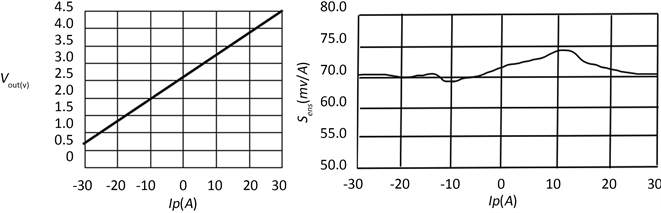(a) (b)

Figure 2. (a) The relationship between the output voltage and the current to be measured; (b) The relationship between sensitivity and current to be measured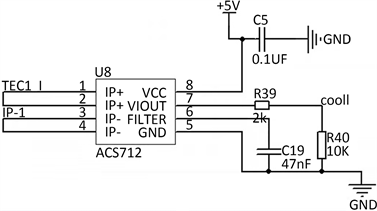Figure 3. Current detection circuit

3.3. 温度传感电路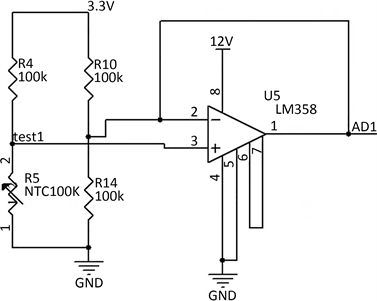Figure 4. Temperature acquisition circuit

3.4. 制冷模块电路

3.5. CAN总线通信

STM32芯片支持CAN通信接口，如图6所示，文中采用电容式隔离CAN总线收发器为ISO1050，此收发器符合或者优于ISO11898-2标准的技术规范，最高工作频率为1 Mbps，为了避免信号的反射与干扰，在信号线之间加120 Ω终端电阻。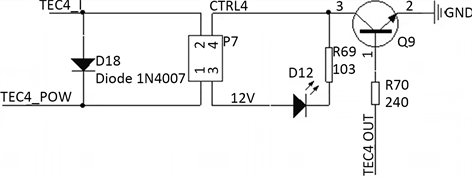Figure 5. Refrigeration module circuit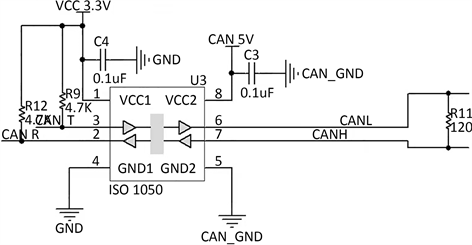Figure 6. CAN communication principle diagram

4. 系统软件单元设计

4.1. 软件设计流程

1) 初始化系统：各使能定时器的初始化，及各初始参数值的设置。

2) 数据采集：该部分程序主要完成由于温度变化而引起的PT100阻值变化，从而输出相应的电压信号；以及在霍尔传感器下检测电流值变化感应输出的电压值变化，并将数据上返给单片机进行相应的数据处理。

3) 继电器控制：主要通过控制直流继电器  进行对制冷片的控制，将继电器的控制引脚进行高低电平置位，从而控制制冷片的工作状态及工作时间，实现间歇式制冷。

4) 数据显示：该部分由数码管进行相应仪器温度及环境温度的显示，以及上位机获取制冷界面的电流值与环境、仪器温度显示。

5) PID控制算法

4.2. PID增量式算法

$U\left(t\right)={K}_{p}\left[e\left(t\right)=1/{T}_{1}\underset{0}{\overset{t}{\int }}e\left(t\right)\text{d}t+{T}_{D}\left(\text{d}e\left(t\right)/\text{d}t\right)\right]$ (1)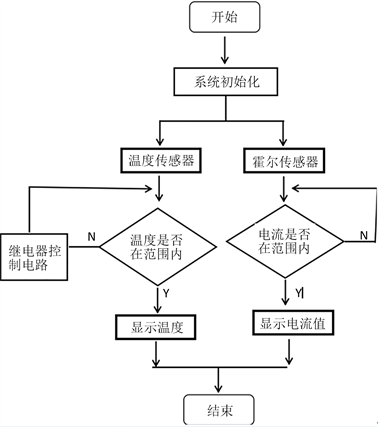Figure 7. System flow chart design

$G\left(s\right)={K}_{p}+\frac{{K}_{p}}{{T}_{1}S}+{K}_{p}{T}_{D}S$ (2)

$\underset{0}{\overset{t}{\int }}e\left(t\right)\text{d}t+{T}_{s}\underset{i=0}{\overset{k}{\sum }}e\left(i\right),\text{\hspace{0.17em}}\frac{\text{d}e\left(t\right)}{dt}=\frac{e\left(k\right)-e\left(k-1\right)}{{T}_{s}}$ (3)

$U\left(k\right)={K}_{p}\left[e\left(k\right)={T}_{s}/{T}_{1}\underset{i=0}{\overset{k}{\sum }}e\left(i\right)+{T}_{D}\left(e\left(k\right)-e\left(k-1\right)\right)/{T}_{s}\right]={K}_{p}e\left(k\right)+{k}_{1}\underset{i=0}{\overset{k}{\sum }}e\left(i\right)+{K}_{D}\left[e\left(k\right)-e\left(k-1\right)\right]$ (4)

{

Pid_Auto.err_last=Pid_Auto.err_next;

Pid_Auto.err_next=Pid_Auto.err; //依次将偏差值赋给上一个

5. 制作与调试

5.1. 实物制作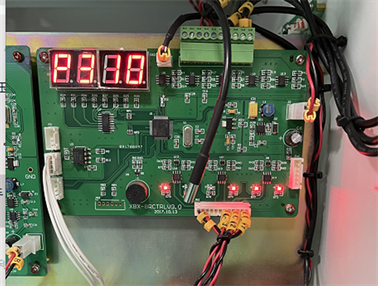Figure 8. Current detection system display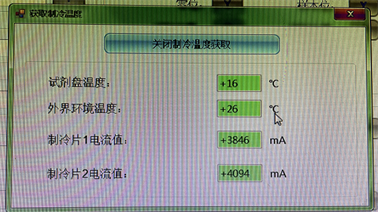Figure 9. Upper computer software design

5.2. 实验数据分析Table 1. Data of measured current values at different times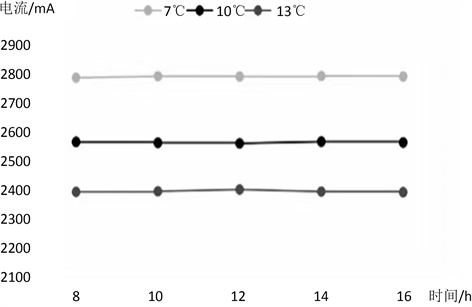Figure 10. Current values measured at different times at the same temperature

6. 结论

 张心怡, 官文亮, 梅政. 电流检测装置[J]. 电子制作, 2018(21): 62-64.

 王仲夏, 章浩, 陈黎明. 基于单片机的电流检测装置的实现[J]. 黑龙江工业学院学报(综合版), 2019, 19(2): 25-28.

 吕英俊, 于佩玲, 张广林, 刘卓伟. 基于STM32的密闭系统温度控制研究[J]. 舰船控制系统, 2018(5): 123.

 刘吉明, 白小峰, 何世安. 基于位置式PID温控系统设计[J]. 环境技术, 2020, 38(6): 1-3.

 戴世纪, 王仲根. 基于Smith预估型模糊PID温度控制系统的设计[J]. 重庆工商大学学报, 2020, 37(6): 2-3.

 杨乔木, 李立伟, 杨玉新, 王凯. 基于STM32的电池管理系统主控单元设计[J]. 电子设计工程, 2021, 29(1): 1-2.

 黄平, 张宇飞. 电流检测装置[J]. 南方农机, 2018, 49(20): 134.

 张铭郎, 郭昭莉, 袁申, 何英昊, 蒋邵君. 基于单片机的电流检测装置[J]. 物联网技术, 2019, 9(5): 2-3.

 郑文豪, 赵鹏, 李刚. 基于单片机的温度控制系统设计[J]. 电子制作, 2019(11): 1-3.

 胡国喜. 半导体制冷技术及应用路径研究[J]. 电子制作, 2020(9): 2.

 苏晨. 基于单片机的数字式通电延时时间继电器设计[J]. 电子技术与软件工程, 2018(18): 232-233.

 李佳升, 熊洁, 阳磊. 基于FFT电流检测装置设计[J]. 湖南城市学院学报, 2020, 29(6): 1-6.

 凌思睿, 曹纯, 郑鑫. 具有副负载电流检测的高速固态继电器接口电路设计[J]. 计算机测量与控制, 2020, 28(9): 1-5.

 佘子敬, 肖程望, 吴建学. 基于PID算法改进的光模块设计与实现[J]. 计算机技术与自动化, 2018, 3(2): 6.

Top